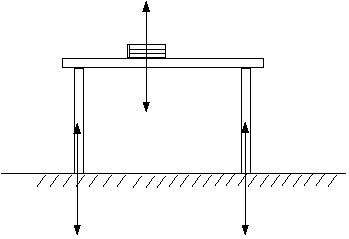## Tuesday, 18 April 2006

### Fisika SMA

Pengalaman Belajar di SMAN BI 1 Banjar

## Newton's Third Law of Motion

SUBJECT: Physics
TOPIC: Force and Motion
DESCRIPTION: A set of mathematics problems dealing with Newton's Laws of Motion.
CONTRIBUTED BY: Carol Hodanbosi
EDITED BY: Jonathan G. Fairman - August 1996

The third law of motion states that if a body exerts a force on a second body, the second body exerts a force that is equal in magnitude and opposite in direction to the first force. So for every action force there is always a reaction force. No force can occur by itself
The book lying on the table is exerting a downward force on the table, while the table is exerting an upward reaction force on the book. Because the forces are equal and opposite, the book remains at rest. Notice also that the table legs are in contact with the floor and exert a force downward on it, while the floor in turn exerts an equal and opposite force upward.Questions for you to consider:

• If forces are always equal and opposite in action and reaction, how is it possible for an object to accelerate?

• Explain, in detail, using the third law of motion, how a person is able to walk forward.